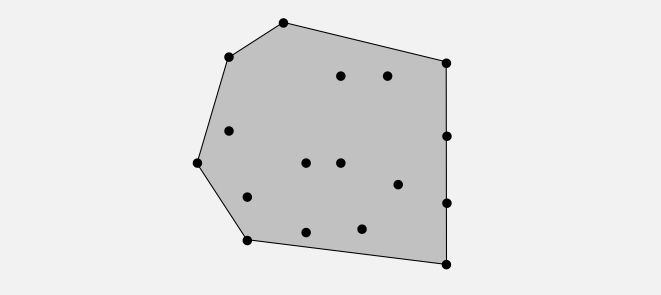• Comparable
• Selection Sort
• Insertion Sort
• Shell Sort
• Shuffle
• Convex hull

# Comparable

Implement the `Comparable` interface to make the `sort()` universal.

``````public class File implements Comparable<File>{
…
public int compareTo(File b){
…
return -1; // this less than b
…
return +1; // this greater than b
…
return 0; // equal
}
…
}
``````
``````public static void sort(Comparable[] a){
int N = a.length;
for (int i = 0; i < N; i++){
for (int j = i; j > 0; j--){
if (a[j].compareTo(a[j-1]) < 0)
exchange(a,j,j-1);
else break; // compare to next
}// end for j
}// end for i
}// end sort()
``````

# Selection sort

• In iteration i, find index `min` of smallest remaining entry.
• Swap a[i] and a[min]
``````public static void sort(Comparable[] a){
int N = a.lengh;
for (int i = 0; i < N; i++){
int min = i;
for (int j = i+1; j < N; j++)
if (less(a[j], a[min]) min = j;
exchange(a, i, min);
}// end for i
}// end sort()
``````

## Facts

• Running time is insensitive to input: always quadratic time
• Data movement is minimal: linear number of exchanges(one-step to its final position)

# Insertion Sort

Animation: Insertion Sort

``````public static void sort(Comparable[] a){
int n = a.length;
// although start with 0 is waste of time, think about if n = 0, 1 will cause mistake, you need another `if` statement to proof it
for (int i = 0; i < n; n++){
for (int j = i; j > 0; j--){
if (less(a[j], a[j-1]) exchange(a, j, j-1);
// all before is already sorted, so do break
else break;
}// end for j
}// end for i
}// end sort()
``````

## Facts

It’s obviously that this sort is highly depend on the data.

• Best case: already in order. N-1 compares, 0 exchange.
• Worst case: reversed order. N(1+N)/2 compares, N(1+N)/2 exchanges. ~1/2 N^2
• Partially-sorted: linear. ~N. This feature will be used in the ‘Shellsort’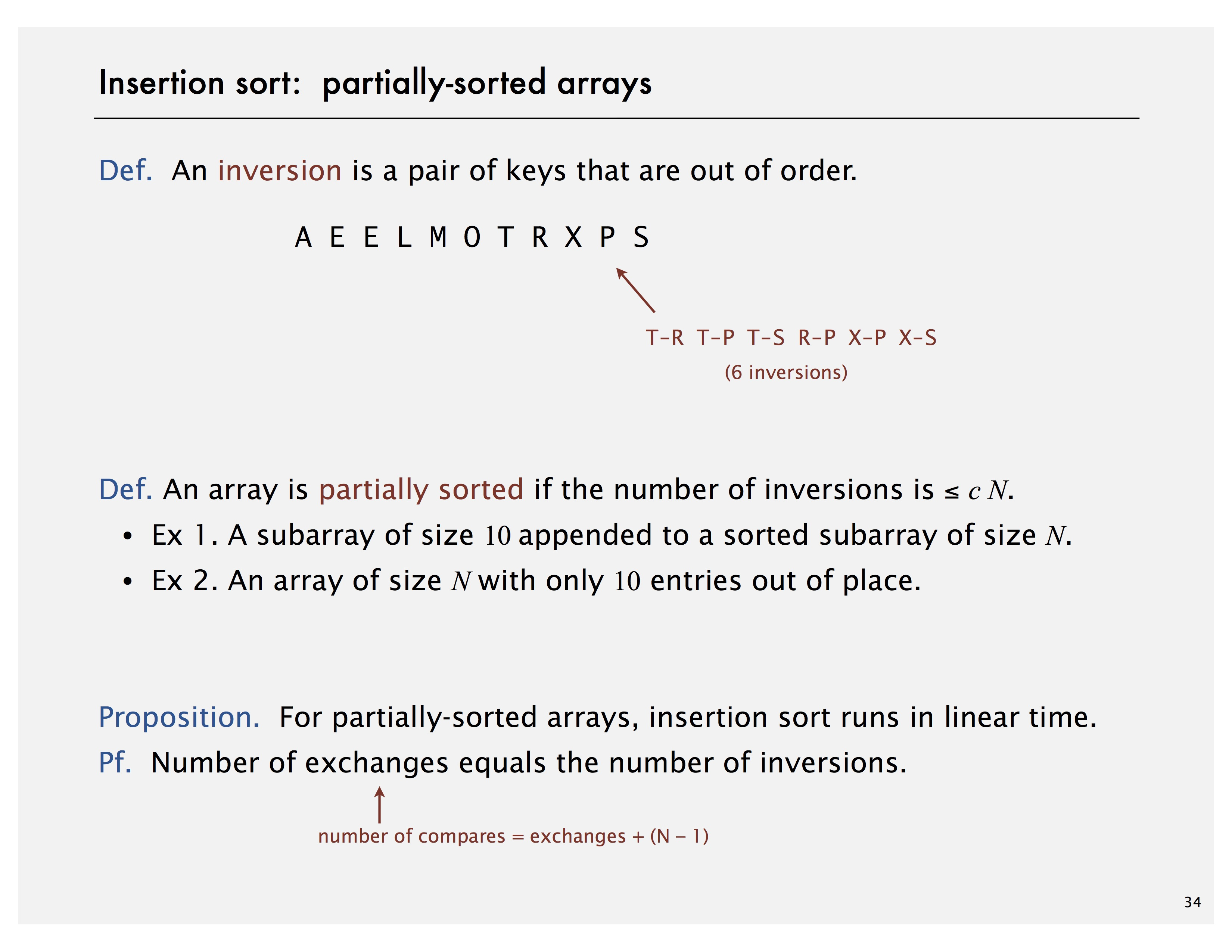# Shellsort

Insertion sort is kind of waste: each time comparing with the the only 1-before item although the `j` might be very small — considering the reverse case.

So we can jump. Compare to k-before item; If bigger, exchange. The result will be `h-sort`. Like this: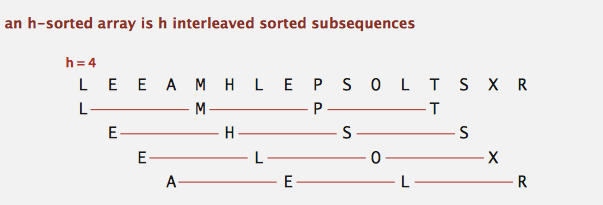every 4 interleaved item is sorted: L, M, P, T; E, H, S, S; … The result will be nearly ordered.

imply `h-sort` with different `h`: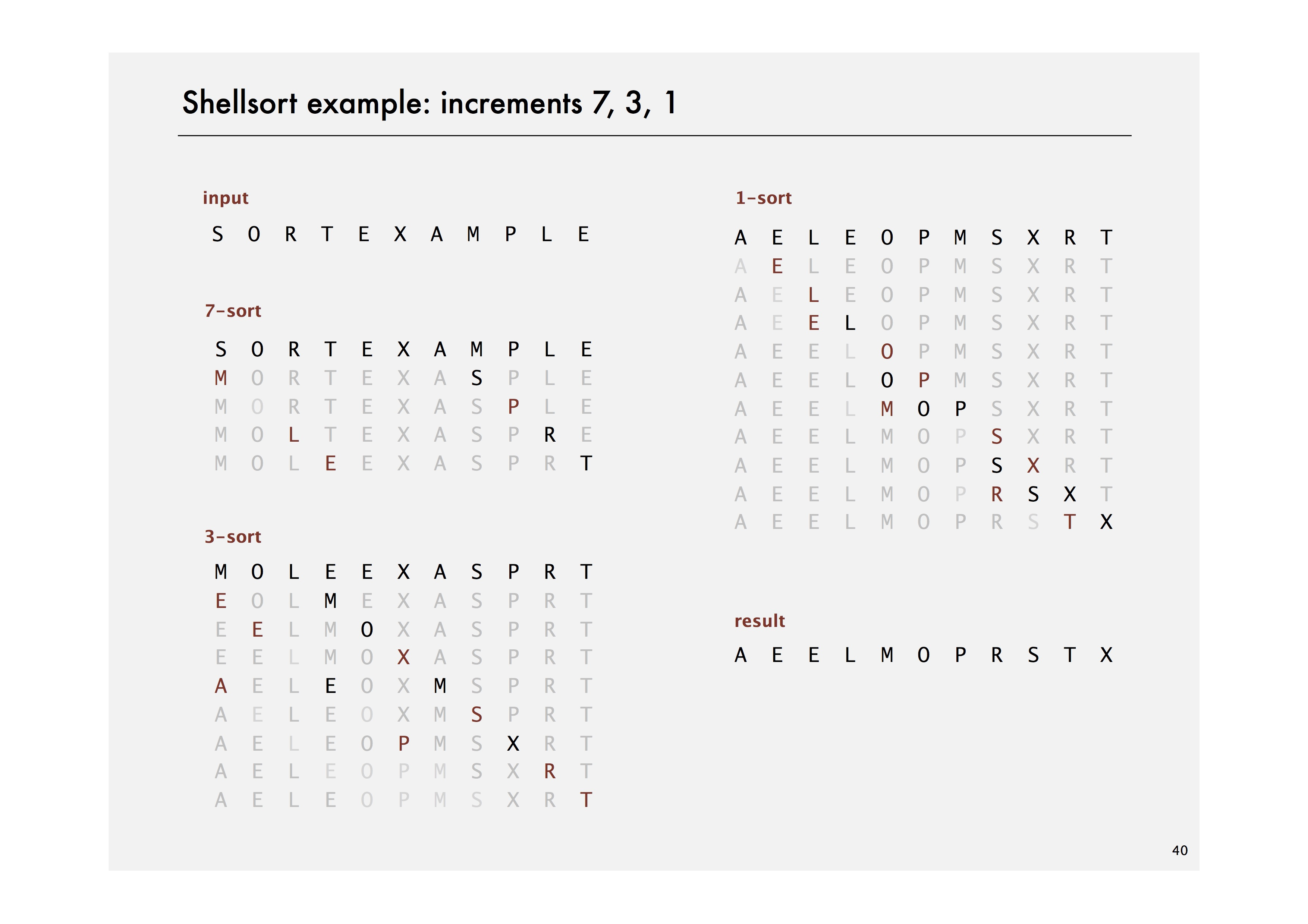A g-sorted array remains g-sorted after h-sorting it.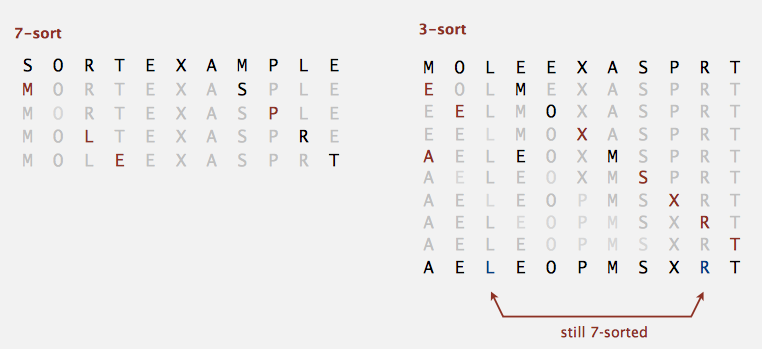It’s quiet nature. I’m not going to proof it..

After 3-sort, we can imply 1-sort, aka, original insertion sort. After that, the array will become sorted.

This algorithm is more efficient. The problem is how to determine the `h`?

• `2^i`: 1, 2, 4, 8, 16, 32, … (BAD: ignore the even position until final 1-sort, the even position may remain very unstable)
• `2^i - 1`: 1, 3, 7, 15, 31, 63, … (MAYBE)
• `3x + 1`(x is the previous one): 1, 4, 13, 40, 121, 364, … (OK)
• Sedgewick (merging of `(9 ⨉ 4 i ) – (9 ⨉ 2 i ) + 1` and `4 i – (3 ⨉ 2 i ) + 1`): 1, 5, 19, 41, 109, 209, 505, 929, 2161, 3905, … (GOOD)

Animation: Shell Sort

## Implement

``````public static void shellSort(Comparable a[]){
int n = a.length;
// in the cycle we do not have another check. we start with h
if (n == 0) return;
int h = 1;
// minium sub-sort array length is 3
while (h < n/3) h = h * 3 + 1;

// until 1-sort
while (h > 0){
for (int i = h; i < n; i++){
for (int j = i; j >= h; j-=h){
if (less(a[j], a[j-h])) exchange(a, j, j-h);
else break;
}// end for j
}// end for i
h /= 3; // h = h / 3; === h = (h - 1) / 3
// 1, 4, 13, 40, 121, 364, ...
}// end while h
}// end sort()
``````

A more beautiful code:

``````for (int j = i; j >= h; j-=h){
if (less(a[j], a[j-h])) exchange(a, j, j-h);
else break;
}// end for j
// === equal to ===
for (int j = i; j >= h && less(a[j], a[j-h]); j -= h)
exchange(a, j, j-h);
``````

we do not need a `else break;` ‘cause the statement is in the rules line.

## Cost

• worst-case with `3x + 1` : O(N^3/2)
• avg with `3x + 1`: NlgN ( it’s a guess, accurate model has not yet been discovered)

Useful in practice:

• Fast unless array size is huge (used for small subarrays).
• Tiny, fixed footprint for code (used in some embedded systems).
• Hardware sort prototype.

Unsolved questions:

• Asymptotic growth rate?
• Best sequence of increments?
• Average-case performance?

## Q: Does prime number is a good increments?

(Q: 素数是不是一个比较好的 increments?) A: No 实验见 Algs-part1-coursera/week-2-stack-queue/prime-shellsort at master · d4rkb1ue/Algs-part1-coursera · GitHub

``````\$ java-algs4 PrimeShellSort < int30000.txt
size: 30000
3x + 1 in 0.014s
prime in 0.109s
\$ java-algs4 PrimeShellSort < int30000.txt
size: 30000
3x + 1 in 0.015s
prime in 0.101s
\$ java-algs4 PrimeShellSort < int30000.txt
size: 30000
3x + 1 in 0.014s
prime in 0.108s
\$ java-algs4 PrimeShellSort < int30000.txt
size: 30000
3x + 1 in 0.015s
prime in 0.104s
``````

# Shuffle

A simply solution is set each item with a random number, and then, base on the giving number to sort these items. However, it’s quiet waste. Sort is very expansive.

``````public void shuffle(Object[] a){
int n = a.length;
for (int i = 0; i < n; i++){
// exchange with [0,i]
exchange(a, i, StdRandom.uniform(i + 1));
}
}
``````

You should exchange with `[0, i]` instead of `[0, n]` because `[i+1, n-1]` is unseen, doing a whole array doesn’t give you a uniformly random result.

## Wrong practice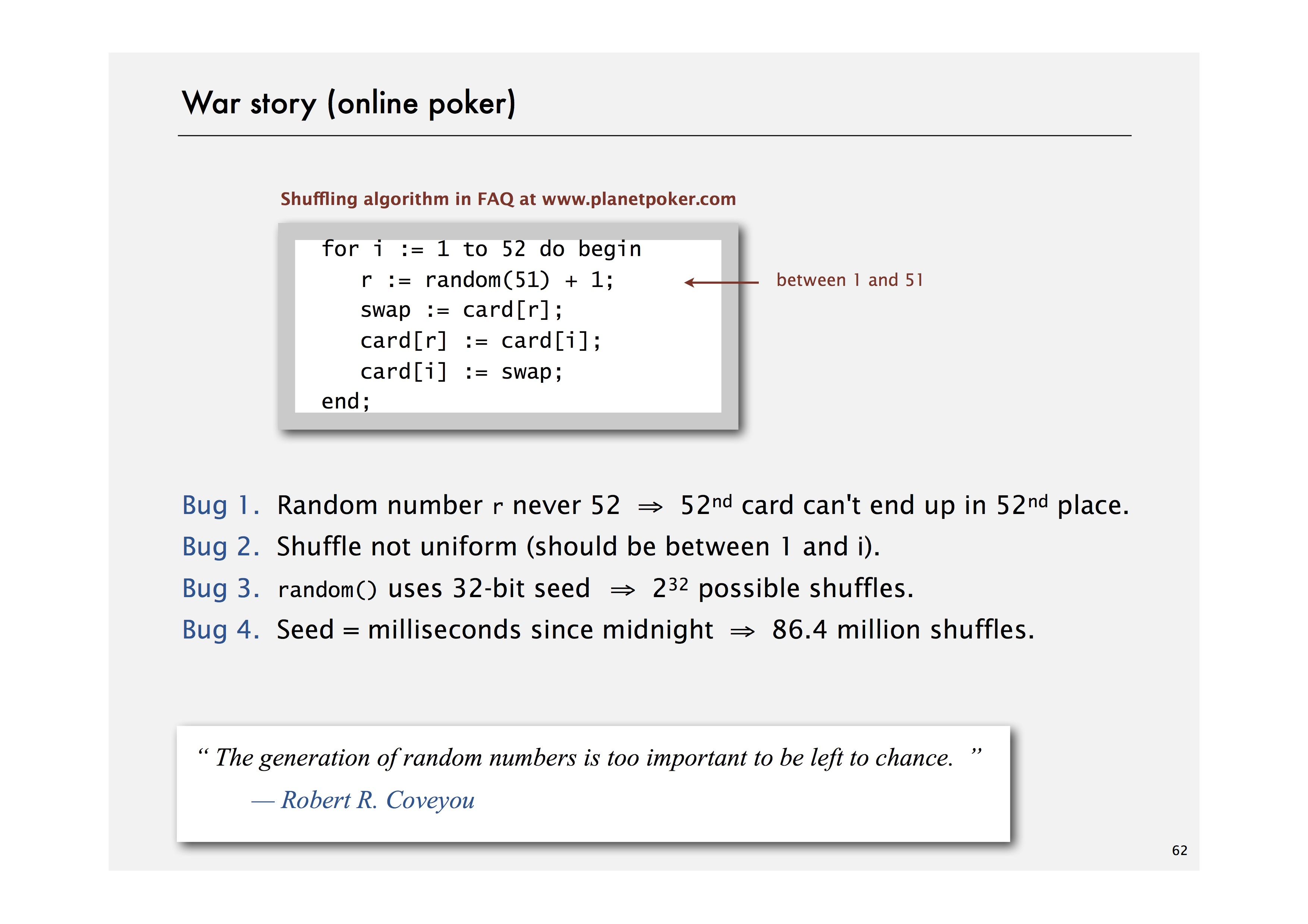# Convex hull

The convex hull of a set of N points is the smallest perimeter fence enclosing the points.